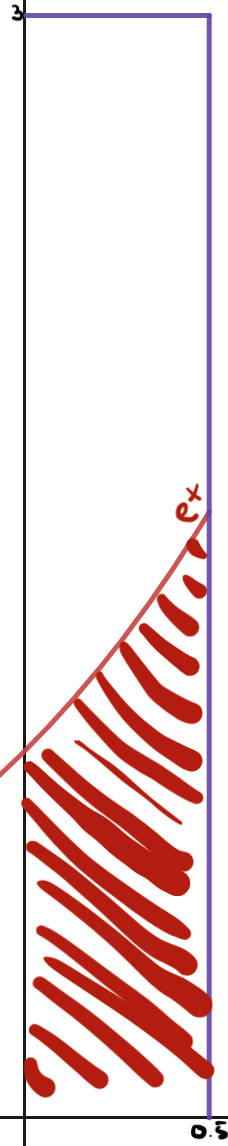# Approximating $$\sqrt{e}$$ Using Everything But $$\sqrt{e}$$

14.1.2023

A fun way to spend in hour is to approximate some number in a silly way. Say we want to approximate $$\sqrt{e}$$, a nice irrational number, but we only know the value of $$e^x$$ for $$x < 0.5$$. Let's suppose also that we can only store one value of $$e^x$$ at a time (so, for instance, we can't just calculate $$e^{1/4} \cdot e^{1/4}$$).

Maybe the simplest way to do this is to just evaluate $$e^x$$ for $$x = 0.499999$$ or something like that. And while that would probably get a pretty decent approximation, it's no fun. Instead, we can be a little more creative :)

Notice that $$\int_0^{0.5} e^x \, dx = \sqrt{e} - 1.$$ So if we can get a decimal approximation of this integral, we can get $$\sqrt{e}$$. So basically we just need to calculate the area under the curve $$f(x) = e^x$$ between $$0$$ and $$0.5$$.So let's approximate the area under this curve with $$0 < x < 0.5$$. We know that $$e^{0.5} < e < 3$$, so let's confine our view of the curve to a 0.5 by 3 box. Now we can do something fun: we can just throw darts at our box. And if we're bad enough at playing darts, we might expect that our darts land randomly and uniformly distributed within our box. That means that, after we throw a lot of darts, we can count them all up and notice the ratio between those that landed in the shaded area and the total thrown. In other words, as we throw more and more darts, $$\frac{\text{# darts in shaded region}}{\text{# total darts}} \approx \frac{\text{area of shaded region}}{\text{total area}} = \frac{\sqrt{e} - 1}{3/2}.$$ So then $$\sqrt{e} \approx \frac{\text{# darts in shaded region} \times 3}{\text{# total darts} \times 2} + 1.$$

So now all we need to do is throw a bunch of darts at the box and count how many land in the shaded region! But, although I'm bad enough at darts to have a decently uniform distribution on the box, my hand starts cramping after a few million throws. So we'll just hire a Python script to do all the work for us1:


SAMPLE = 10000000

for i in range(SAMPLE):
x = random.uniform(0.0, 0.5)
y = random.uniform(0.0, 3)

if y < math.e ** x:

The most popular way of approximating $$e$$, especially in setting world records for longest approximation, is through approximating the Taylor series expansion $$\sum_{j=0}^\infty \frac{1}{j!}$$ with some added optimizations. Even with a quick unoptimized script on my Raspberry Pi, this method can match the true value of $$e$$ to as many decimal places a Python float can store with just the first 20 terms of the Taylor series and within a few seconds. And indeed we can apply this to $$\sqrt{e}$$ by either applying a square root algorithm to the previously calculated $$e$$ or by calculating $$\sum_{j=0}^\infty \frac{(1/2)^j}{j!}$$. Either way, this would converge much much faster to $$e$$ than the nondeterministic Monte Carlo method above. And so for all practical purposes, it's probably not useful at all to calculate this constant probabilistically, but who cares it was fun :)
1. Okay so technically the random.uniform function in Python includes both arguments in the range, and in particular means that it's possible for $$0.5$$ to be chosen as value for $$x$$. But nothing would really change if there were a (more appropriate) function that excluded its arguments in the context of this problem.